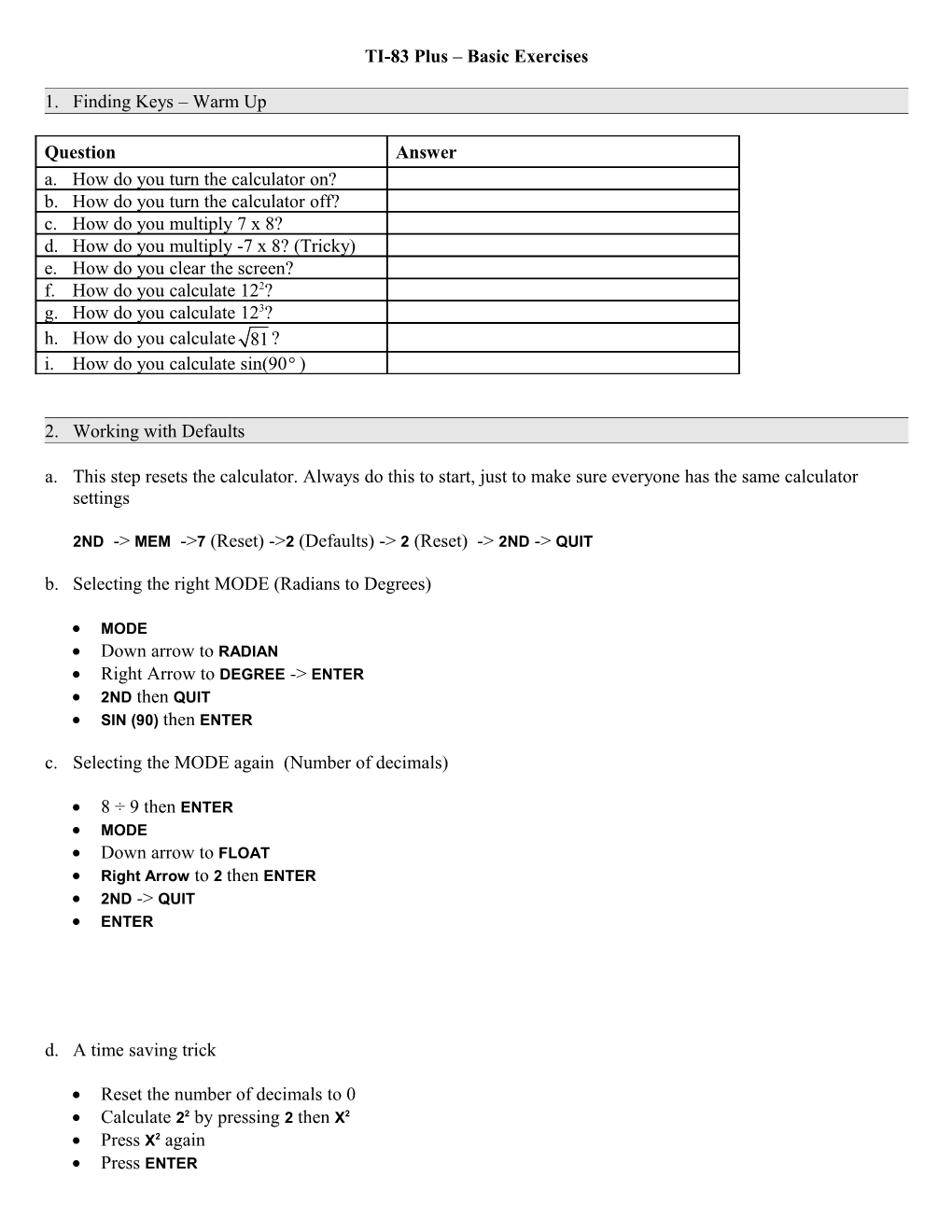# TI83 Plus Basic ExercisesTI-83 Plus – Basic Exercises

1. Finding Keys – Warm Up

1. How do you turn the calculator on?

1. How do you turn the calculator off?

1. How do you multiply 7 x 8?

1. How do you multiply -7 x 8? (Tricky)

1. How do you clear the screen?

1. How do you calculate 122?

1. How do you calculate 123?

1. How do you calculate?

1. How do you calculate sin(90)

1. Working with Defaults
1. This step resets the calculator. Always do this to start, just to make sure everyone has the same calculator settings
2ND -> MEM ->7 (Reset) ->2 (Defaults) -> 2(Reset) -> 2ND -> QUIT
2. Selecting the right MODE (Radians to Degrees)
• MODE
• Right Arrow to DEGREE -> ENTER
• 2ND thenQUIT
• SIN (90) thenENTER
1. Selecting the MODE again (Number of decimals)
• 8 ÷ 9 then ENTER
• MODE
• Down arrow to FLOAT
• Right Arrow to 2 thenENTER
• 2ND -> QUIT
• ENTER
1. A time saving trick
• Reset the number of decimals to 0
• Calculate22 by pressing 2 thenX2
• Press X2 again
• Press ENTER
• Press ENTER
• Press ENTER
1. Simple Graphing
1. y = x
• Press Clear
• Press Y= (blue keys just under screen)
• Press X,T,θ,n key (just under the Mode key)
• Press Graph key (light grey keys just under screen)
1. y = x +2
• Press Clear
• Press Y=key
• Press Downarrow (to enter equation for Y2)
• Press X,T,θ,n then + 2
• Press Graph key (light grey keys just under screen)
1. y = x2
• Press Clear
• Press Y= key
• Press Down arrow twice (to enter equation for Y3)
• Press X,T,θ,n then X2
• Press Graphkey(blue keys just under screen)
1. Selecting graphs (say you just want the y = x2 graph, but you don’t want to erase the other formulas)
1. Press Y= key
• Cursor should be flashing on the first X in the Y1 equation
• Press the left cursor so it is over the equals sign
1. Press ENTER
• Press down arrow so it is over the equals sign in th4e next equation
1. Press ENTER
2. Press down arrow (notice that the equals signs are not highlighted in the first 2 equations (this means they won’t be displayed when you press the GRAPH key)
3. Press GRAPH key
1. Turning off the calculator and coming back
1. Press 2ND then ON
2. PressON
• Press Y=
• Notice that your equations are still there
• This is why reset the defaults when you start a class
1. Press CLEAR
1. More Complex Calculations

52 – (-3)3 +

1. 5
2. X2
3. – (minus sign, not negative sign)
4. (
5. – (negative sign)
6. 3
7. )
8. MATH (find math key)
9. 3
10. +
11. 4
12. MATH
13. 5
14. (
15. 2
16. ^
17. 8
18. )
19. ENTER

1. Using Variables

Which coordinate pair yields the greatest value in the expression 2x2 -3xy –y2?

(1,4) (3,2) (5,0)

1. CLEAR
2. 1
3. STO (find this key)
4. X,T,θ,n
5. ENTER
6. 4
7. STO
8. APLHA (find this key)
9. 1
10. ENTER
11. 2
12. X,T,θ,n
13. x2
14. – (minus sign, not negative)
15. 3
16. X,T,θ,n
17. APLHA
18. 1
19. APLHA
20. 1
21. x2
22. ENTER
23. You should get the answer -26

Now lets enter (3,2) into the equation 2x2 -3xy –y2

1. 3
2. STO
3. X,T,θ,n
4. ENTER
5. 2
6. STO
7. ALPHA
8. 1
9. Instead of typing in the whole equation, lets retrieve it
10. 2ND
11. Enter (this bring up last entry)
12. 2ND
13. Enter(this bring second last entry)
14. 2ND
15. Enter (this brings up the equation we want)
16. You should get an answer of -4

Finally lets enter (5,0) into the equation 2x2 -3xy –y2

1. 5
2. STO
3. X,T,θ,n
4. ENTER
5. 0
6. STO
7. ALPHA
8. 1
9. 2ND
10. Enter (this bring up last entry)
11. 2ND
12. Enter(this bring second last entry)
13. 2ND
14. Enter (this brings up the equation we want)
15. You should get an answer of 50

the coordinates (5,0) yield the largest value in the equation 2x2 -3xy –y